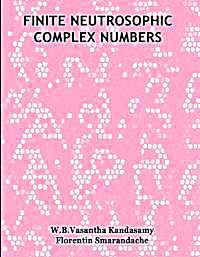#jsDisabledContent { display:none; } My Account | Register | Help

### AlgebraAlgebra is the branch of mathematics concerning the study of the rules of operations and relations, and the constructions and concepts arising from them, including terms, polynomials, equations and algebraic structures. Together with geometry, analysis, topology, combinatorics, and number theory, algebra is one of the main branches of pure mathematics.•### An Elementary Treatise on Logarithms : With Tables of the Logarithms

##### By: Webster Wells
•### Tables for the Formation of Logarithms and Anti-Logarithms to Twen...

##### By: Peter Gray
•### Challenging complex number problems : IIT JEE Complex Numbers (part 1)

##### By: Sal Khan

2010 IIT JEE Paper 1 Problem 39 Complex Numbers (part 1)

Understanding i and the complex plane

•### Multiplying monomials, binomials and polynomials in general : Fact...

##### By: Sal Khan, Monterey Institute for Technology and Education

Factoring and the Distributive Property 2

Using polynomial expressions and factoring polynomials.

•### Multiplying monomials, binomials and polynomials in general : Fact...

##### By: Sal Khan, Monterey Institute for Technology and Education

Factoring and the Distributive Property

Using polynomial expressions and factoring polynomials.

•### Challenging complex number problems : IIT JEE Complex Numbers (part 3)

##### By: Sal Khan

2010 IIT JEE Paper 1 Problem 39 Complex Numbers (part 3)

Understanding i and the complex plane

•### Completing the square and the quadratic formula : Discriminant of ...

##### By: Sal Khan, CK-12 Foundation

Exploring quadratics and higher degree polynomials. Also in-depth look at rational functions.

•### Multiplying monomials, binomials and polynomials in general : Fact...

##### By: Sal Khan, Monterey Institute for Technology and Education

Factoring and the Distributive Property 3

Using polynomial expressions and factoring polynomials.

•### Challenging complex number problems : IIT JEE Complex Numbers (part 2)

##### By: Sal Khan

2010 IIT JEE Paper 1 Problem 39 Complex Numbers (part 2)

Understanding i and the complex plane

•### Finite Neutrosophic Complex Numbers

##### By: Florentin Smarandache; W. B. Vasantha Kandasamy

This book is organized into 5 chapters. The first chapter introduces real neutrosophic complex numbers. Chapter two introduces the notion of finite complex numbers; algebraic structures like groups, rings etc are defined using them. Matrices and polynomials are constructed using these finite complex numbers. Chapter three introduces the notion of neutrosophic complex modulo integers. Algebraic structures using neutrosophic complex modulo integers are built and around 90 ...

In this chapter we for the first time we define the notion of integer neutrosophic complex numbers, rational neutrosophic complex numbers and real neutrosophic complex numbers and derive interesting properties related with them.

•### Complex numbers : Example: Complex roots for a quadratic

##### By: Sal Khan, Monterey Institute for Technology and Education

Complex Roots from the Quadratic Formula

Understanding and solving equations with imaginary numbers. . Conceptual videos and worked examples from basic algebra through algebra 2. Includes videos from the former algebra worked examples playlists.

•### Overview and history of algebra : Origins of Algebra

##### By: Sal Khan

Where did the word Algebra and its underlying ideas come from

Videos exploring why algebra was developed and how it helps us explain our world.

•### Algebra II : Algebra II: Imaginary and Complex Numbers

##### By: Sal Khan

23-26, mostly imaginary and complex numbers

Sal works through California Standards Test problems. Whether you are in California and studying for that test or just want additional practice, this is a good place to review the major math concepts.

•### Algebra II : Algebra II: Complex numbers and conjugates

##### By: Sal Khan

27-31, complex numbers and conjugates

Sal works through California Standards Test problems. Whether you are in California and studying for that test or just want additional practice, this is a good place to review the major math concepts.

•### Ratios with algebra : More advanced ratio problem--with Algebra (H...

##### By: Sal Khan

More advance ratio problem with algebra.

You have probably been wondering whether our powers of algebraic problem solving break down if we divide by the variable or we have entire expressions in denominator of a fraction. Well, they don't! In this topic, you'll learn how to interpret and manipul

•### Ratios with algebra : Ratio problem with basic algebra (new HD)

##### By: Sal Khan

A slightly more involved ratio problem with algebra

You have probably been wondering whether our powers of algebraic problem solving break down if we divide by the variable or we have entire expressions in denominator of a fraction. Well, they don't! In this topic, you'll learn how to interpret and manipul

•### Overview and history of algebra : The Beauty of Algebra

##### By: Sal Khan

Why the abstraction of mathematics is so fundamental

Videos exploring why algebra was developed and how it helps us explain our world.

•### Algebra II : CA Standards: Algebra II

##### By: Sal Khan

13-16 (polynomial factoring and multiplication)

Sal works through California Standards Test problems. Whether you are in California and studying for that test or just want additional practice, this is a good place to review the major math concepts.

•### Algebra II : Algebra II: Simplifying Polynomials

##### By: Sal Khan

17-22, simplifying polynomials and algebraic expressions

Sal works through California Standards Test problems. Whether you are in California and studying for that test or just want additional practice, this is a good place to review the major math concepts.

•### Complex numbers : Complex Conjugates Example

##### By: Sal Khan, Monterey Institute for Technology and Education

Complex Conjugates

Understanding i and the complex plane